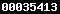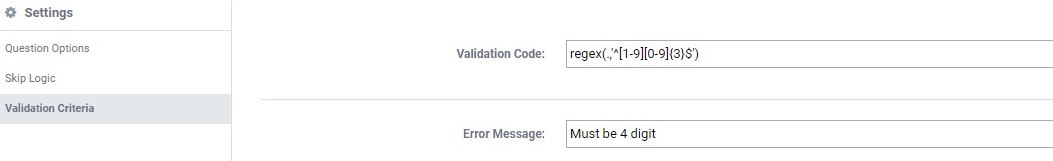Validation codes in KoBoToolbox, a wonderful feature to restrict variables

# Commonly used validation codes in KoBoToolbox

## What are validation codes in KoBoToolbox?

KoBoToolbox has a wonderful feature to restrict any variable under certain validation criteria. However, this is known as the validation code in KoBoToolbox. Suppose you want to restrict the Age variable under the validation criteria that Age will lie in a range of between 15 and 45. That means Age is always greater than 14 and less than 46.

Therefore, KoBoToolbox allows you to set these types of validation criteria using appropriate validation codes. Today, we are going to discuss some commonly used validation codes in KoBoToolbox.

## How to set validation criteria?

In general, there are two ways to set validation criteria in KoBoToolbox: 1) Using the Validation Criteria option of Settings in KoBoToolbox edit, or 2) Using the Constraint field in Excel file downloaded from KoBoToolbox.

In the first place, in KoBoToolbox edit, there are two boxes to set validation criteria. One is the Validation Code box and the other is Error Message box. Eventually, the Validation Code box is for putting the validation code as well as the Error Message box is for putting the error message.

On the other hand, in an Excel file, there are two fields to set validation criteria. One is the constraint field and the other is the constraint_message field. Eventually, the constraint field is for putting the validation code as well as the constraint_message field is for putting the error message.

## Some validation codes with explanations

Nevertheless, the below table shows some validation codes as well as their appropriate explanations:

 Validation code Explanation regex(.,’^[1-9][0-9]{3}\$’) The response must be of 4 digit where the 1st digit lies between 1 to 9; and 2nd, 3rd, and 4th digits lie between 0 to 9. regex(.,’^[0-9]{4}\$’) The response must be of 4 digits where all digits lie between 0 to 9. regex(., ‘^[0-0][0-9][0-9][0-9][0-9][0-9][0-9][0-9][0-9][0-9][0-9]\$’) This is the response for 11 digit cell number where the 1st digit must be 0, and the remaining 10 digits lie between 0 to 9. In this case, the variable type must be text. . > 14 and . < 46 The response must lie between 15 to 45.

In conclusion, these are some few examples of validation codes in KoBoToolbox. Furthermore, you can look into KoBoToolbox Help Center for other validation codes.

SummaryArticle Name
Commonly used validation codes in KoBoToolbox
Description
KoBoToolbox has a wonderful feature to restrict any variable under certain validation criteria. However, this is known as validation codes in KoBoToolbox. Suppose you want to restrict the Age variable under the validation criteria that Age will lie in a range of between 15 and 45. That means Age is always greater than 14 and less than 46.
Author
Publisher Name
LEARN AND EARN
Publisher Logo